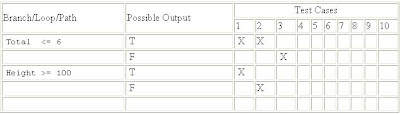## Sunday, April 19, 2009

### What is Branch Testing?

Branch Testing

Testing designed to execute each outcome of each decision point in a computer program [IEEE610]. For every decision point in the code, each branch is chosen at least once.

Branch Testing Example
`declare Height as integer declare Total as integer         GET Height;         GET Total;         WHILE (Total <= 6) LOOP             IF (Height >= 100) THEN                 Height = Height - 2;             ELSE                 Height = Total * Height;             END IF             Total = Total + 1;         END;         PRINT Height; `Test CasesMore Examples of Branch Testing

This example has two branches to be executed:
`IF ( x equals y) THEN    statement oneELSE    statement twoEND IF`

This examples also has just two branches to be executed, despite the compound condition:
`IF ( x equals y AND a greater than b ) THEN    statement oneELSE    statement twoEND IF`

Also See:
Statement Coverage

Path Testing

Basis Path Testing